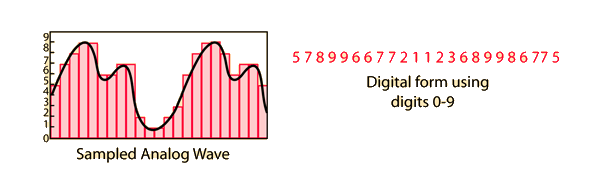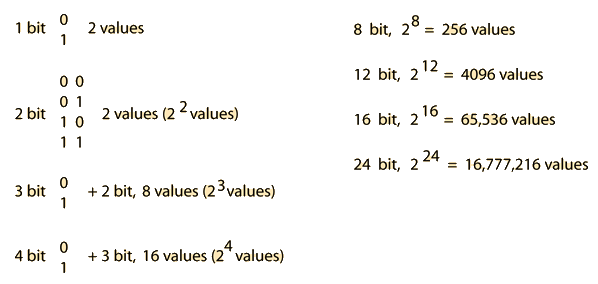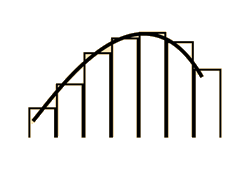# Digital Sampling

For the purpose of storing audio information in digital form, like a compact disc, the normal continuous wave audio signal (analog) must be converted to digital form (analog-to-digital) conversion. Below is an example of a D/A conversion using digits 0-9, but practical schemes store the numbers in binary form. The number of bits in the binary sampler determines the accuracy with which the analog signal can be represented in digital form.From this crude picture of digitizing in steps, perhaps you can appreciate the industry standard of 16 bit sampling in which the voltage is sampled into 65,536 steps. In addition to the number of steps, the rate of sampling also affects the fidelity of representation of the analog waveform. The standard sampling rate is 44.1 kHz, so the assignment of one of 65, 536 values to the signal is done 44,100 times per second. If this recorded data is read from the CD in real time, you are processing 1.4 million bits of information per second (1.4 Mbps).

 MP3 Digital
Index

Traveling wave concepts

Sound reproduction concepts

Audio signal concepts

 HyperPhysics***** Sound R Nave
Go Back

# Implication of Number of Bits

The number of bits used in a digital sampler determines the resolution of the "image" of the signal being represented. A one-bit sampler would be like a light switch, having two possible values, on and off. Samplers with multiple bits are representing a signal in terms of a binary number, and the number of bits determines the number of values which can be expressed, just as a 3 digit number in the usual 10-based number system can take more values than a 2 digit number. The number of bits determines the possible dynamic range.Index

Sound reproduction concepts

Audio signal concepts

 HyperPhysics***** Sound R Nave
Go Back

# Bits and Dynamic Range

If you are doing a linear stair-step digitization of an analog waveform, the number of bits used in the sampling determines the maximum dynamic range you can faithfully represent with the sampling.For example, an 8-bit sample can give you 256 possible values, so if the softest sound you sample is represented by a 1, then the loudest sound you can represent is a 256. The maximum range of amplitudes is then 256, and since the power is proportional to the amplitude squared, the power range is 256 squared or 1 to 65,536 in power. This corresponds to a dynamic range of 48 decibels whereas the dynamic range of an orchestra is about 40 to 100 dB, or 60 dB. By similar calculation, a 12 bit digitization can give you a dynamic range of 72 dB and 16 bits can give you 96 dB of dynamic range.
Index

Sound reproduction concepts

Audio signal concepts

 HyperPhysics***** Sound R Nave
Go Back